# Gyrogroup implies left-inverse property loop

## Statement

Suppose$(G,*)$ is a gyrogroup with neutral element$e$ and inverse map${}^{-1}$. Then,$(G,*)$ is a left inverse property loop with the same neutral element$e$ and where the left inverse map is the same as the inverse map.

## Proof

Given: A gyrogroup$(G,*)$, elements$a,b \in G$.

To prove: There exists unique$u \in G$ such that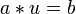$a * u = b$ and there exists unique$y \in G$ such that$v * a = b$ (together, these prove it's a loop). Further,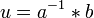$u = a^{-1} * b$ (this shows the left inverse property).

Proof:

Step no. Assertion/construction Facts used Given data used Previous steps used Explanation
1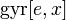$\operatorname{gyr}[e,x]$ is the identity map for all$x \in G$. We note that, for any$y \in G$,$e * (x * y) = x * y = (e * x) * y$. By uniqueness of$\operatorname{gyr}[e,x]y$, we get$\operatorname{gyr}[e,x]y = y$.
2 Suppose$x,z \in G$ are such that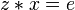$z * x = e$. Then,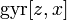$\operatorname{gyr}[z,x]$ is the identity map. gyroassociativity Step (1) By the left loop property,$\operatorname{gyr}[z,x] = \operatorname{gyr}[z * x,x] = \operatorname{gyr}[e,x]$ which is the identity map by Step (1).
3$\operatorname{gyr}[a^{-1},a]$ is equal to the identity map. Step (2)
4 We have$a * u = b \implies a^{-1} * (a * u) = a^{-1} * b$. This simplifies to$u = a^{-1} * b$. Step (3) By Step (3), we obtain that$a^{-1} * (a * u) = (a^{-1} * a) * u$ and thus it simplifies to$e * u = u$.
5$v * a = b \implies \operatorname{gyr}[v,a] = \operatorname{gyr}[b,a]$ left loop property we use that$\operatorname{gyr}[v,a] = \operatorname{gyr}[v * a,a]$
6 We have$v * a = b \implies v = b * \operatorname{gyr}[b,a](a^{-1})$. In particular,$v$ exists and is uniquely determined by$a,b$. Step (5) Consider the gyroassociativity of$v,a,a^{-1}$:$v * (a * a^{-1}) = (v * a) * \operatorname{gyr}[v,a]a^{-1}$. Simplifying the left side gives$v = (v * a) * \operatorname{gyr}[v,a]a^{-1}$. Now use Step (5) and we are done.

Steps (4) and (6) complete the proof.